# Voltage Follower OP Amplifier: What is it? (Gain & Circuit Diagram)

Contents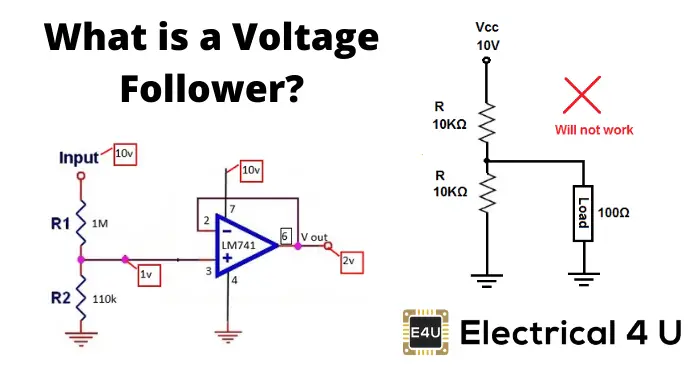## What is a Voltage Follower?

A voltage follower (also known as a buffer amplifier, unity-gain amplifier, or isolation amplifier) is an op-amp circuit whose output voltage is equal to the input voltage (it “follows” the input voltage). Hence a voltage follower op-amp does not amplify the input signal and has a voltage gain of 1.

The voltage follower provides no attenuation or amplification—only buffering.

A voltage follower circuit has a very high input impedance. This characteristic makes it a popular choice in many different types of circuits that require isolation between the input and output signal.

The circuit of voltage follower is shown below.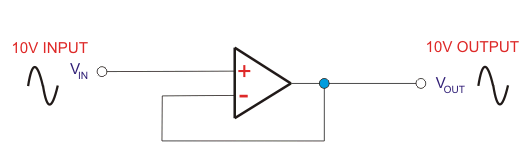An important law that underpins a voltage follower is Ohm’s law.

Which states that a circuit’s current is equal to its voltage divided by its resistance.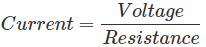As mentioned, voltage followers have a very high input impedance (and hence high resistance).

But before we discuss circuits with high impedance, it will be helpful to first understand what goes on in a circuit with a low impedance.

A low input impedance—and hence resistance in this case—will result in the “R” in the formula for Ohm’s law being small.

With a fixed voltage (V), this will mean that a large amount of current will be drawn by a low-impedance (resistance) load.

Hence the circuit takes a large amount of power from the power source, resulting in high source disturbances.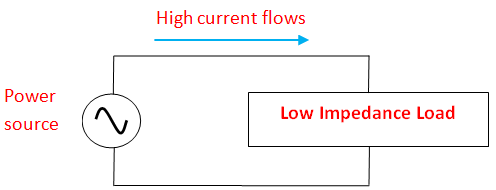Now let’s consider giving the same power to a voltage follower circuit.

A voltage follower circuit is shown below.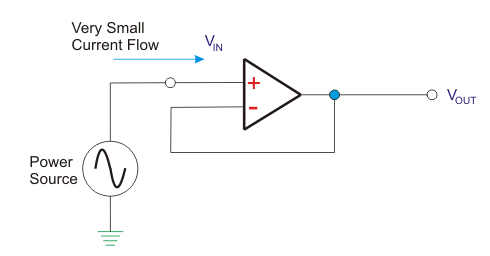Notice how the output is connected to its inverting input.

This connection forces the op-amp to adjust its output voltage to equal the input voltage.

The output voltage hence “follows” the input voltage.

As mentioned, a voltage follower is a type of op-amp with a very high impedance.

More specifically, the input side of the op-amp has a very high impedance (1 MΩ to 10 TΩ), while the output does not.

Now Ohm’s law still needs to hold true.

So if we keep the voltage the same on the input and output side, and we significantly drop the resistance… what is going to happen to the current?

That’s right: the current skyrockets.

A voltage follower keeps the voltage the same—we didn’t say it kept the current the same too!

While a voltage follower has unity voltage gain (i.e. it equals one), it has a very high current gain.

So on the input side: very high impedance, and very low current.

And on the output side: very low impedance, and very high current.

Voltage stays the same, but the current goes up (because impedance went down between the input and the output side).

As mentioned: the input impedance of the op-amp is very high (1 MΩ to 10 TΩ).

With such a high input impedance, the op-amp does not load down the source and draws only minimal current from it.

Because the output impedance of the op-amp is very low, it drives the load as if it were a perfect voltage source.

Both the connections to and from the buffer are therefore bridging connections.

This results in reduced power consumption in the source, and less distortion from overloading and other causes of electromagnetic interference.

## Voltage Follower Gain

A voltage follower has a voltage gain of 1 (unity), because the output voltage follows the input voltage. Although the voltage gain of a voltage buffer amplifier is approximately unity, it provides considerable current and power gain. Despite this it is common to say that it has a gain of 1—referring to the voltage gain (the equivalent 0 dB).

## Voltage Follower in Voltage Divider Circuits

In every circuit, voltage is shared or distributed to the impedance or resistance of the connected components. When an op-amp is connected, a large voltage drop across it will occur due to its high impedance.

So, if we use voltage follower in voltage divider circuits, it will let adequate voltage to be supplied across the load.

Let us go through a voltage divider circuit with a voltage follower, as shown in the figure below.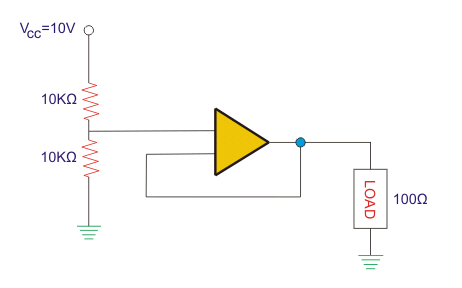Here, the voltage divider is in the middle of two 10 KΩ resistors and the Op-amp. This Op-amp will offer input resistance of some hundreds of megaohm. Now, we can assume it to be 100 MΩ. So the equivalent parallel resistance will be 10 KΩ || 100 KΩ.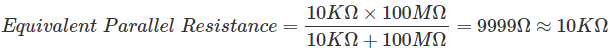So, we get 10KΩ || 10KΩ. We know that the voltage divider, which comprises two similar resistances, will offer exactly half of the power source voltage.

We can prove it using the voltage divider formula as follows: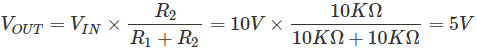Thus, this 5V will drop across the 10KΩ resistance in the top and 5V drop across the resistance 10KΩ in the bottom and the load resistance 100Ω (since 10 KΩ||100 Ω, the same voltage will drop in resistors which are in parallel).

We have seen how the Op-amp works as a buffer for getting the desired voltage to the connected load. In the same circuit with the absence of a voltage follower, it will not work due to the lack of sufficient voltage across the load.

Mainly, the voltage follower is implemented in circuits for two reasons. One is isolating purpose, and the other is for buffering the output voltage from an electrical or electronic circuit to get the desired voltage to the connected load.

The advantages of voltage followers include:

• Provides power gain and current gain.
• Low output impedance to the circuit, which uses the output of the voltage follower.
• The Op-amp takes zero current from the input.

## Applications of Voltage Followers

Some of the applications of voltage followers include:

• Buffers for logic circuits.
• In Sample and hold circuits.
• In Active filters.
• In Bridge circuits via a transducer.

Want To Learn Faster? 🎓
Get electrical articles delivered to your inbox every week.
No credit card required—it’s 100% free.